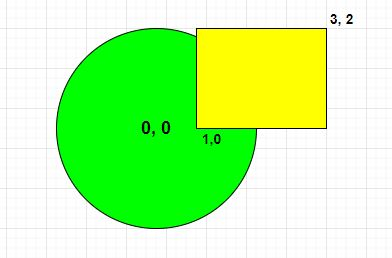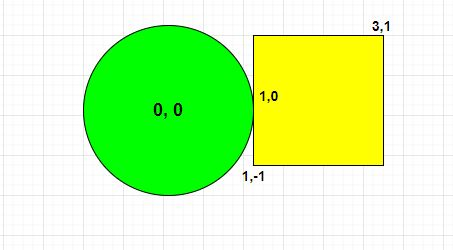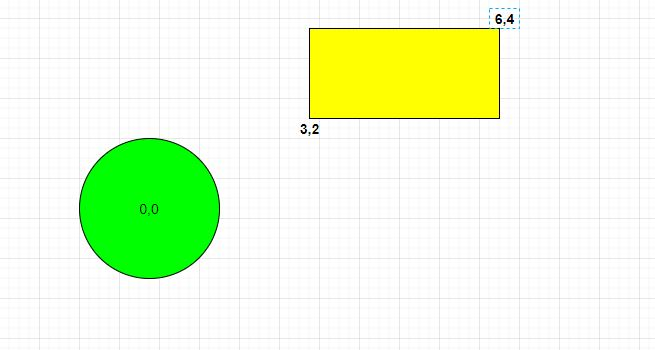Problem title
Difficulty
Avg time to solve

N Queue Using Array
Easy
15 mins
Find a value in BST
Easy
15 mins
Stack Using Deque
Easy
15 mins
Generate All Valid Strings
Easy
15 mins
Raid In The City
Easy
15 mins
Minimum Connection Changes
Moderate
30 mins
Substring Permutation
Moderate
15 mins
Ninja And Alien
Hard
45 mins
Chocolates With Magical Number
Easy
10 mins
Hide And Seek
Moderate
20 mins3

# METEOR GARDEN

Difficulty: EASY
Avg. time to solve
15 min
Success Rate
85%

Problem Statement

#### Note :

``````If any point of the circle lies on the rectangle or inside the rectangle we say the circle is overlapping the rectangle.
``````

#### Input Format :

``````The first line contains an integer 'T' which denotes the number of test cases or queries to be run.

The first line of each test case contains seven space-separated integers ‘xC’, ‘yC’, ‘RAD’, ‘X1’, ‘Y1’, ‘X2’, ‘Y2’ where ‘xC’ and ‘yC’ represents the center coordinates of a circle and ‘RAD’ represents the radius of the circle and ‘X1’, ‘Y1’ represents the coordinates of the bottom left corner and ‘X2’, ‘Y2’ represents the coordinates of the top right corner of the rectangle respectively.
``````

#### Output Format :

``````For each test case, print a single line containing ‘True’ if the meteor overlaps with the garden else, print ‘False’.
The output of each test case will be printed in a separate line.
``````

#### Note :

``````You do not need to print anything, it has already been taken care of. Just implement the given function.
``````

#### Constraints :

``````1 <= T <= 5
-10^4 <= xC, yC, X1, Y1, X2, Y2 <= 10^4
X1 < X2, Y1 < Y2

Where ‘T’ is the number of test cases, ‘xC’ and ‘yC’ represents the center coordinates of a circle and ‘RAD’ represents the radius of the circle and ‘X1’, ‘Y1’ represents the coordinates of the bottom left corner and ‘X2’, ‘Y2’ represents the coordinates of the top right corner of the rectangle.

Time Limit: 1 sec
``````

#### Sample Input 1 :

``````2
0 0 2 1 0 3 2
0 0 1 1 -1 3 1
``````

#### Sample Output 1 :

``````True
True
``````

#### Explanation for sample input 1 :

``````Test Case 1 :
````````````As you can observe the radius of the circle is ‘2’ and according to their coordinates, we can say they will collapse so we return ‘True’ as the answer.

Test Case 2 :
````````````As you can see in the picture there is a common point (1, 0) that lies both on the circle and rectangle so we return ‘True’ for this case.
``````

#### Sample Input 2 :

``````1
0 0 1 3 2 6 4
``````

#### Sample Output 2 :

``````False
``````

#### Explanation for sample input 2 :

``````Test Case 1:
````````````As you can observe, rectangles and circles have no common points so we can say they will not collapse so we return ‘False’ as the answer.
``````Console